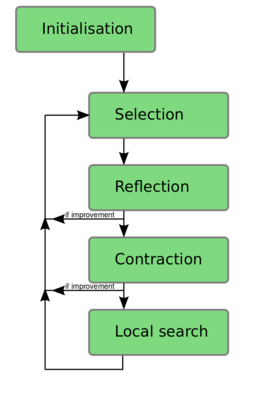# Sandbox

(diff) ← Older revision | Latest revision (diff) | Newer revision → (diff)

## Math test

Use LaTeX syntax.

Warning: the Preview doesn't work for mathematical expressions. You have to Publish (or Save page).

$N=20*\sqrt{D}$

$$\operatorname{erfc}(x) = \frac{2}{\sqrt{\pi}} \int_x^{\infty} e^{-t^2}\,dt = \frac{e^{-x^2}}{x\sqrt{\pi}}\sum_{n=0}^\infty (-1)^n \frac{(2n)!}{n!(2x)^{2n}}$$

$$N=\max\left(40+2\sqrt{D},\sqrt{40^{2}+\left(D+2\right)^{2}}\right)\tag{1}$$

## Text + Image MapThe main idea was to define ...

...

...

...